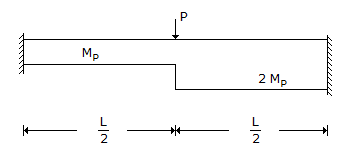# Civil Engineering - UPSC Civil Service Exam Questions

36.

The optimistic, most likely and pessimistic time estimates of an activity are 5, 10 and 21 days respectively. What are the expected time and standard deviation respectively ?

 A. 12, 3 B. 11, 4 C. 11, 2.67 D. 10, 16

Explanation:

No answer description available for this question. Let us discuss.

37.

A fixed beam made of steel is shown in the figure. At collapse, the value of load P will be equal to :A. 10 Mp/L B. 12 Mp/L C. 16 Mp/L D. 20 Mp/L

Explanation:

No answer description available for this question. Let us discuss.

38.

Consider the following statements :
The use of relatively weak motor
1. will accommodate movements due to loads, cracking if any and will be distributed as thin hair cracks which are less noticeable or harmful.
2. will result in reduction of stresses due to differential expansion of masonry units.
Of these statements :

 A. 1 alone is correct B. 2 alone is correct C. both 1 and 2 are correct D. neither 1 nor 1 is correct

Explanation:

No answer description available for this question. Let us discuss.

39.

A stone dropped from the top of a tower is found to travel (5/9) of the height of the tower during the last second of its fall. What is the total time of fall ?

 A. 2 s B. 3 s C. 6 s D. 4 s

Explanation:

No answer description available for this question. Let us discuss.

40.

Field vane shear is the appropriate field test for obtaining the shear strength of which one of the following ?

 A. Stiff clay B. Weathered rock C. Sand D. Soft clay